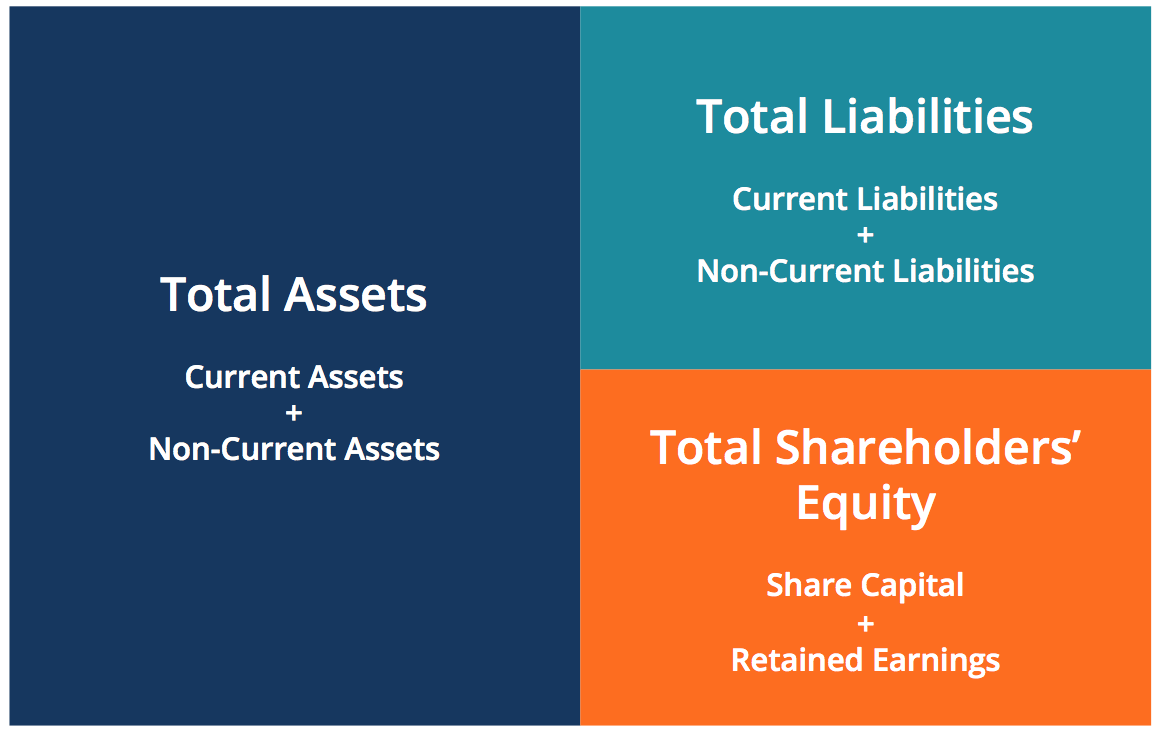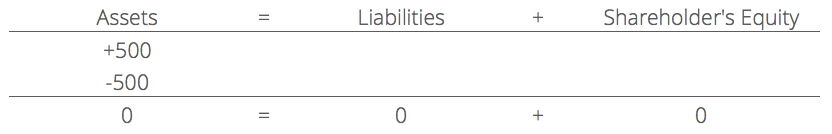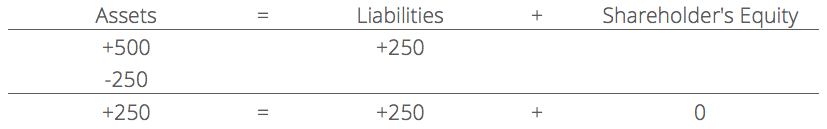# Accounting Equation

The basis of accounting.

## What is the Accounting Equation?

The accounting equation is a basic principle of accounting and a fundamental element of the balance sheet. The equation is as follows:

Assets = Liabilities + Shareholder’s Equity

This equation sets the foundation of double-entry accounting and highlights the structure of the balance sheet. Double-entry accounting is a system where every transaction affects both sides of the accounting equation. For every change to an asset account, there must be an equal change to a related liability or shareholder’s equity account. It is important to keep the accounting equation in mind when performing journal entries.

The balance sheet is broken down into three major sections and their various underlying items: Assets, Liabilities, and Shareholder’s Equity.

Learn to read a balance sheet and other financial statements with CFI’s reading financial statements course!Below are some examples of items that fall under each section:

• Assets: Cash, Accounts Receivable, Inventory, Equipment
• Liabilities: Accounts Payable, Short-term borrowings, Long-term Debt
• Shareholder’s Equity: Share Capital, Retained Earnings

The accounting equation shows the relationship between these items.

### Rearranging the Accounting Equation

The accounting equation can also be rearranged into the following form:

Shareholder’s Equity = Assets – Liabilities

In this form, it is easier to highlight the relationship between shareholder’s equity and debt (liabilities). As you can see, shareholder’s equity is the remainder after liabilities has been subtracted from assets. This is because creditors – parties that lend money – have the first claim to a company’s assets.

For example, if a company becomes bankrupt, its assets are sold and these funds are used to settle debts first. Only after debts are settled are shareholders entitled to any of the company’s assets to attempt to recover their investments.

Regardless of how the accounting equation is represented, it is important to remember that the equation must always balance.

### Examples of the Accounting Equation

For every transaction, both sides of this equation must have an equal net effect. Below are some examples of transactions and how they affect the accounting equation.

#### 1. Purchasing a Machine with Cash

Company XYZ wishes to purchase a \$500 machine using only cash. This transaction would result in a credit to Equipment (+\$500) and a debit to Cash (-\$500). The net effect on the accounting equation would be as follows:This transaction affects only the assets of the equation, therefore there is no corresponding effect in liabilities or shareholder’s equity in the right side of the equation.

#### 2. Purchasing a Machine with Cash and Credit

Company XYZ wishes to purchase a \$500 machine but it only has \$250 of cash in its holdings. The company is allowed to purchase this machine with an initial payment of \$250 but it owes the manufacturer the remaining amount. This would result in a credit to Equipment (+\$500), a credit to Accounts Payable (+\$250) and a debit to Cash (-\$250). The net effect on the accounting equation would be as follows:This transaction affects both sides of the accounting equation; both the left and right side of the equation increase by +\$250.

• Accounting Fundamentals